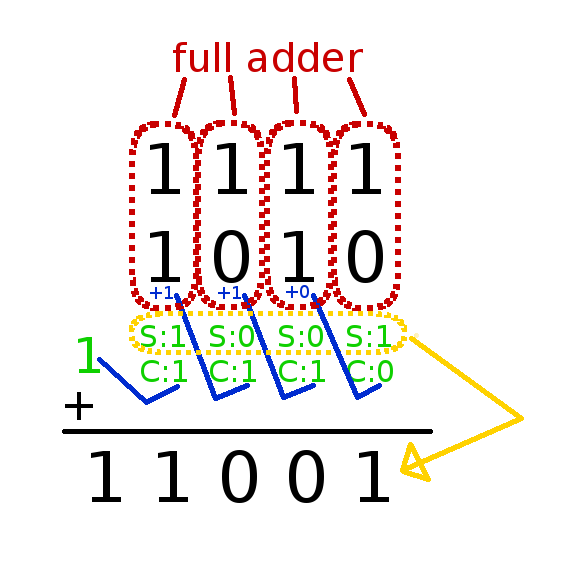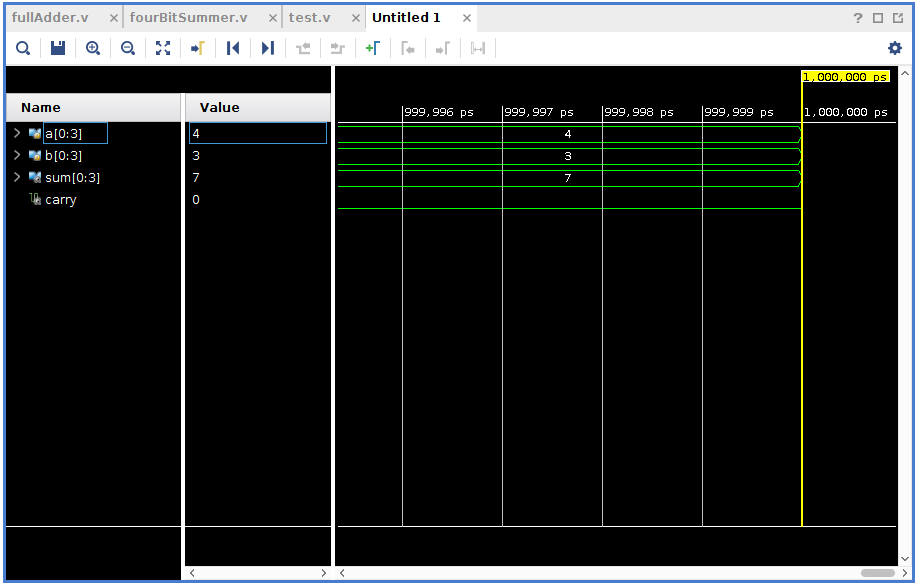You know how to do addition using step-by-step. Always, the carry value is add to next step and the sum value is written to result. I tried to tell these in a picture:If we want to write an algorithm for these, how to do this?

 1. Variables Inputs: a (4 bit), b (4 bit) Outputs: sum (4 bit), carry (1 bit) Others: carryValuesFromFullAdders (5 bit) (With this, we store carry values and use this value next step. Always, first bit is 0, because of there isn’t any operation before first bit pair so there is no ‘carry in’ value.) 2. Full Adder for Every Bit Pair Use full adder step-by-step for bit pairs, so when finish last step, we have final result.

Verilog code for the algorithm:

1. Full Adder Code (We Need! You can use another codes like the previous blog post):

```
input a,
input b,
input carryIn,
output sum,
output carryOut
);
assign {carryOut,sum}=carryIn+a+b;
endmodule

```

```
module fourBitSummer(
input [0:3] a,
input [0:3] b,
output [0:3] sum,
output carry
);

wire [0:4] oncekiBasamaktanEldeKalan;
assign oncekiBasamaktanEldeKalan=0;

genvar sayac;
generate
for(sayac=0;sayac<4;sayac=sayac+1)
begin: basamakBasamakTopla
end
endgenerate
assign carry=oncekiBasamaktanEldeKalan;
endmodule

```

Bonus – Test Code:

```
module test(

);

reg [0:3] a;
reg [0:3] b;

wire [0:3] sum;
wire carry;

fourBitSummer test(a,b,sum,carry);

initial
begin
#10 a=4'b0100; b=4'b0011;
end

endmodule

```

Here, test result 🙂